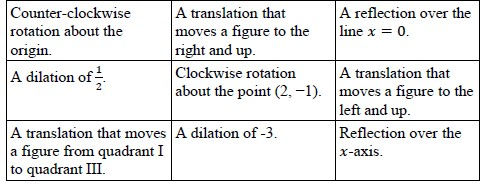# MA.912.GR.2.2Export Print
Identify transformations that do or do not preserve distance.

### Clarifications

Clarification 1: Transformations include translations, dilations, rotations and reflections described using words or using coordinates.

Clarification 2: Instruction includes recognizing that these transformations preserve angle measure.

General Information
Subject Area: Mathematics (B.E.S.T.)
Strand: Geometric Reasoning
Status: State Board Approved

## Benchmark Instructional Guide

### Terms from the K-12 Glossary

• Coordinate Plane
• Dilation
• Origin
• Reflection
• Rigid Transformation
• Rotation
• Scale Factor
• Translation

### Vertical Alignment

Previous Benchmarks

Next Benchmarks

### Purpose and Instructional Strategies

In grade 8, students were introduced to transformations and whether they preserve congruence or similarity. In Geometry, students determine which transformations preserve distance (rigid transformations, or rigid motions) and which do not (non-rigid transformations, or nonrigid motions), while understanding that all the transformations in this benchmark preserve angle measures. This discussion leads to the definition of congruence and the definition of similarity in terms of rigid and non-rigid motions. (MTR.3.1, MTR.5.1) In later courses, other non-rigid motions are studied, including stretches in one coordinate direction.
• Instruction includes the comparison of a variety of geometric figures before and after a single transformation (including reflections, translations, rotations and dilations) to solidify that each of these transformations preserves angle measure. (MTR.4.1
• Instruction includes the student understanding that in order for a transformation based on stretching and shrinking to preserve angle measure, it must have a stretch or shrink of the same scale factor in both the $x$-direction and the $y$-direction.
• For example, the transformation ($x$, $y$) → (2.5$x$, 2.5$y$) would preserve angle measure, but the transformation ($x$, $y$) → (2.5$x$, 3.5$y$) would not.
• While the intent of the benchmark is to focus on dilations, instruction includes the introduction of other non-rigid transformations to showcase that not all non-rigid transformations preserve angle measure.
• For example, “stretch” or “shrink” in the direction of one of the axes, such as ($x$, $y$) → (5$x$, $y$) or ($x$, $y$) → ($x$, $\frac{\text{y}}{\text{6}}$), does not preserve angle measure. Students can visualize this using geometric software to see how each affects angle measures of a triangle.
• Transformations should be presented using words and using coordinates.
• Instruction includes the use of folding paper (e.g., patty paper) for hands-on experiences, as needed, and interactive geometry software to allow more flexibility in exploration, when possible.

### Common Misconceptions or Errors

• Students may incorrectly assume that dilations change angle measures.
• Students may assume that dilations only occur in either the $x$-direction or the $y$-direction based on knowledge of function transformations.
• Students may assume that every non-rigid motion preserves angle measures since the only non-rigid motions in the benchmark are dilations, which do preserve angle measures. But many non-rigid motions preserve neither length nor angle measure.

• Penelope made the following statement in Geometry class, “Figure A is a rotation of Figure B about the origin.” Chalita disagreed because distance and angle measures are not preserved between the two figures.
• Figure A has the coordinate points (1, −1), (3, −1) and (1, −2).
• Figure B has the coordinate points (0.8, 1), (1, 3) and (2, 0.8).
• Part A. What does “distance and angle measures are not preserved” mean in relation to the two figures?
• Part B. Determine whether angle measures were preserved from Figure A to Figure B.
• Part C. Determine whether distance measures were preserved from Figure A to Figure B.
• Part D. Based on your answers from Part B and Part C, determine which student is correct.

• Sort the following transformations into preserves distance and does not preserve distance.### Instructional Items

Instructional Item 1
• Circle the transformations that can be used when it is important to preserve angle measure.
Horizontal Translations Reflections Clockwise Rotations
Dilations Vertical Translations Counterclockwise Rotations

Instructional Item 2
• Circle the transformations that can be used when it is important to preserve distance.
Horizontal Translations Reflections Clockwise Rotations
Dilations Vertical Translations Counterclockwise Rotations

Instructional Item 3
• Write a transformation, or sequence of transformations, that preserves angle measure but does not preserve distance.

*The strategies, tasks and items included in the B1G-M are examples and should not be considered comprehensive.

## Related Courses

This benchmark is part of these courses.
1200400: Foundational Skills in Mathematics 9-12 (Specifically in versions: 2014 - 2015, 2015 - 2022, 2022 and beyond (current))
1206310: Geometry (Specifically in versions: 2014 - 2015, 2015 - 2022, 2022 and beyond (current))
1206320: Geometry Honors (Specifically in versions: 2014 - 2015, 2015 - 2022, 2022 and beyond (current))
1206315: Geometry for Credit Recovery (Specifically in versions: 2014 - 2015, 2015 - 2022, 2022 and beyond (current))
7912065: Access Geometry (Specifically in versions: 2015 - 2022, 2022 and beyond (current))

## Related Access Points

Alternate version of this benchmark for students with significant cognitive disabilities.
MA.912.GR.2.AP.2: Select a transformation that preserves distance.

## Related Resources

Vetted resources educators can use to teach the concepts and skills in this benchmark.

## Formative Assessment

Comparing Transformations:

Students are asked to determine whether or not dilations and reflections preserve distance and angle measure.

Type: Formative Assessment

## MFAS Formative Assessments

Comparing Transformations:

Students are asked to determine whether or not dilations and reflections preserve distance and angle measure.

## Student Resources

Vetted resources students can use to learn the concepts and skills in this benchmark.

## Parent Resources

Vetted resources caregivers can use to help students learn the concepts and skills in this benchmark.